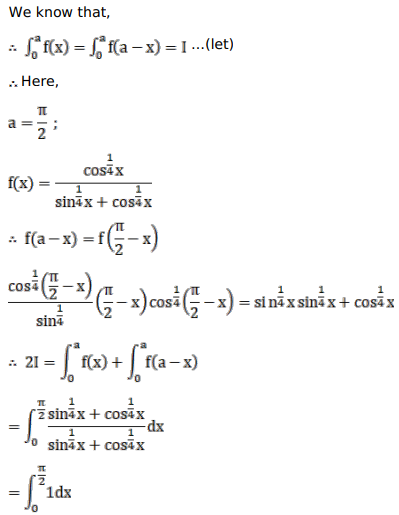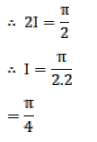# Mark against the correct answer in the following:

Question:

Mark $(\sqrt{ })$ against the correct answer in the following:

$\int_{0}^{\pi / 2} \frac{\cos ^{1 / 4} x}{\left(\sin ^{1 / 4} x+\cos ^{1 / 4} x\right)} d x=?$

A. 0

B. 1

C. $\frac{\pi}{4}$

D. none of these

Solution: